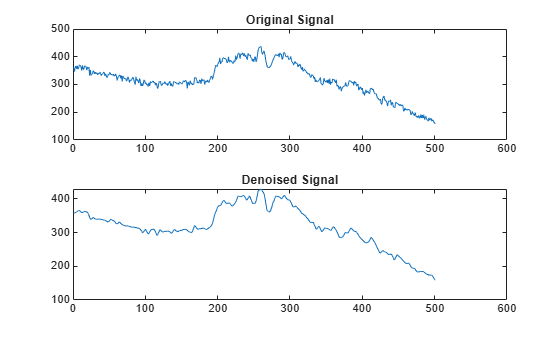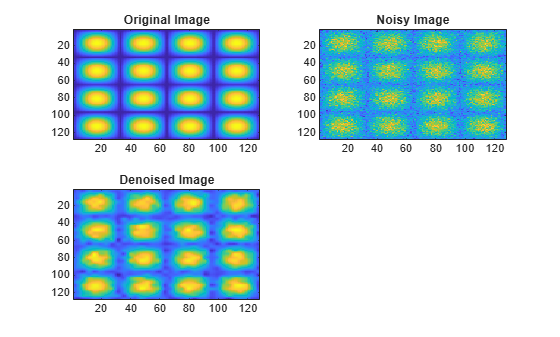Documentation

# wdencmp

Denoising or compression

## Syntax

``[XC,CXC,LXC,PERF0,PERFL2] = wdencmp('gbl',X,wname,N,THR,SORH,KEEPAPP)``
``[___] = wdencmp('gbl',C,L,wname,N,THR,SORH,KEEPAPP)``
``[___] = wdencmp('lvl',X,wname,N,THR,SORH)``
``[___] = wdencmp('lvl',C,L,wname,N,THR,SORH)``

## Description

example

````[XC,CXC,LXC,PERF0,PERFL2] = wdencmp('gbl',X,wname,N,THR,SORH,KEEPAPP)` returns a denoised or compressed version `XC` of the input data `X` obtained by wavelet coefficients thresholding using the global positive threshold `THR`. `X` is a real-valued vector or matrix. [`CXC`,`LXC`] is the `N`-level wavelet decomposition structure of `XC` (see `wavedec` or `wavedec2` for more information). `PERFL2` and `PERF0` are the L2-norm recovery and compression scores in percentages, respectively. If `KEEPAPP` = 1, the approximation coefficients are kept. If `KEEPAPP` = 0, the approximation coefficients can be thresholded.```
````[___] = wdencmp('gbl',C,L,wname,N,THR,SORH,KEEPAPP)` uses the wavelet decomposition structure [`C`,`L`] of the data to be denoised or compressed.```
````[___] = wdencmp('lvl',X,wname,N,THR,SORH)` uses the level-dependent thresholds `THR`. The approximation coefficients are kept.```
````[___] = wdencmp('lvl',C,L,wname,N,THR,SORH)` uses the wavelet decomposition structure [`C`,`L`].```

## Examples

collapse all

Denoise 1-D electricity consumption data using the Donoho-Johnstone global threshold.

Load the signal and select a segment for denoising.

```load leleccum; indx = 2600:3100; x = leleccum(indx);```

Use `ddencmp` to determine the default global threshold and denoise the signal. Plot the original and denoised signals.

```[thr,sorh,keepapp] = ddencmp('den','wv',x); xd = wdencmp('gbl',x,'db3',2,thr,sorh,keepapp); subplot(211) plot(x); title('Original Signal'); subplot(212) plot(xd); title('Denoised Signal');```Denoise an image in additive white Gaussian noise using the Donoho-Johnstone universal threshold.

Load an image and add white Gaussian noise.

```load sinsin Y = X+18*randn(size(X));```

Use `ddencmp` to obtain the threshold.

`[thr,sorh,keepapp] = ddencmp('den','wv',Y);`

Denoise the image. Use the order 4 Symlet and a two-level wavelet decomposition. Plot the original image, the noisy image, and the denoised result.

```xd = wdencmp('gbl',Y,'sym4',2,thr,sorh,keepapp); subplot(2,2,1) imagesc(X) title('Original Image') subplot(2,2,2) imagesc(Y) title('Noisy Image') subplot(2,2,3) imagesc(xd) title('Denoised Image')```## Input Arguments

collapse all

Input data to denoise or compress, specified by a real-valued vector or matrix.

Data Types: `double`

Wavelet expansion coefficients of the data to be compressed or denoised, specified as a real-valued vector. If the data is one-dimensional, `C` is the output of `wavedec`. If the data is two-dimensional, `C` is the output of `wavedec2`.

Example: ```[C,L] = wavedec(randn(1,1024),3,'db4')```

Data Types: `double`

Size of wavelet expansion coefficients of the signal or image to be compressed or denoised, specified as a vector or matrix of positive integers.

For signals, `L` is the output of `wavedec`. For images, `L` is the output of `wavedec2`.

Example: ```[C,L] = wavedec(randn(1,1024),3,'db4')```

Data Types: `double`

Name of wavelet, specified as a character vector or string scalar, to use for denoising or compression. See `wavemngr` for more information. `wdencmp` uses `wname` to generate the `N`-level wavelet decomposition of `X`.

Level of wavelet decomposition, specified as a positive integer.

Threshold to apply to the wavelet coefficients, specified as a scalar, real-valued vector, or real-valued matrix.

• For the case `'gbl'`, `THR` is a scalar.

• For the one-dimensional case and `'lvd'` option, `THR` is a length `N` real-valued vector containing the level-dependent thresholds.

• For the two-dimensional case and `'lvd'` option, `THR` is a 3-by-`N` matrix containing the level-dependent thresholds in the three orientations: horizontal, diagonal, and vertical.

Data Types: `double`

Type of thresholding to perform:

• `'s'` — Soft thresholding

• `'h'` — Hard thresholding

See `wthresh` for more information.

Threshold approximation setting, specified as either `0` or `1`. If `KEEPAPP = 1`, the approximation coefficients cannot be thresholded. If ```KEEPAPP = 0```, the approximation coefficients can be thresholded.

Data Types: `double`

## Output Arguments

collapse all

Denoised or compressed data, returned as a real-valued vector or matrix. `XC` and `X` have the same dimensions.

Wavelet expansion coefficients of the denoised or compressed data `XC`, returned as a real-valued vector. `LXC` contains the number of coefficients by level.

Size of wavelet expansion coefficients of the denoised or compressed data `XC`, specified as a vector or matrix of positive integers. If the data is one-dimensional, `LXC` is a vector of positive integers (see `wavedec` for more information). If the data is two-dimensional, `LXC` is a matrix of positive integers (see `wavedec2` for more information).

Compression score, returned as a real number. `PERF0` is the percentage of thresholded coefficients that are equal to 0.

`PERFL2` = 100 * (vector-norm of `CXC` / vector-norm of `C`)2 if `[C,L]` denotes the wavelet decomposition structure of `X`.

If `X` is a one-dimensional signal and `'wname'` an orthogonal wavelet, `PERFL2` is reduced to

`$\frac{100{‖XC‖}^{2}}{{‖X‖}^{2}}$`

## Algorithms

The denoising and compression procedures contain three steps:

1. Decomposition.

2. Thresholding.

3. Reconstruction.

The two procedures differ in Step 2. In compression, for each level in the wavelet decomposition, a threshold is selected and hard thresholding is applied to the detail coefficients.

 DeVore, R. A., B. Jawerth, and B. J. Lucier. “Image Compression Through Wavelet Transform Coding.” IEEE Transactions on Information Theory. Vol. 38, Number 2, 1992, pp. 719–746.

 Donoho, D. L. “Progress in Wavelet Analysis and WVD: A Ten Minute Tour.” Progress in Wavelet Analysis and Applications (Y. Meyer, and S. Roques, eds.). Gif-sur-Yvette: Editions Frontières, 1993.

 Donoho, D. L., and I. M. Johnstone. “Ideal Spatial Adaptation by Wavelet Shrinkage.” Biometrika. Vol. 81, pp. 425–455, 1994.

 Donoho, D. L., I. M. Johnstone, G. Kerkyacharian, and D. Picard. “Wavelet Shrinkage: Asymptopia?” Journal of the Royal Statistical Society, series B, Vol. 57, No. 2, pp. 301–369, 1995.

 Donoho, D. L., and I. M. Johnstone. “Ideal denoising in an orthonormal basis chosen from a library of bases.” C. R. Acad. Sci. Paris, Ser. I, Vol. 319, pp. 1317–1322, 1994.

 Donoho, D. L. “De-noising by Soft-Thresholding.” IEEE Transactions on Information Theory. Vol. 42, Number 3, pp. 613–627, 1995.

##### Support평가판 신청